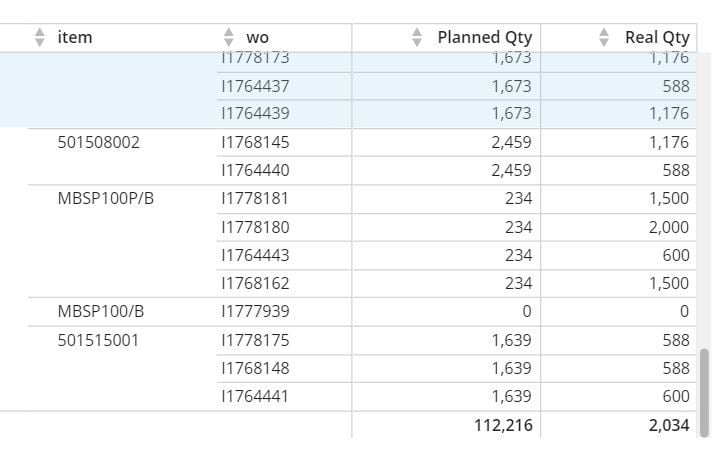# Beast Mode - forEach

Hello. So I have this dataset where I get item, work order and the quantities both planned and actual. This is to calculate manufacturing absorption %.

Is it possible, via beast mode, to tell the function that for each distinct work order, it sums its quantity and displays it as the total of Actual production?

If I do an AVG of the real Qty, it does get the original work order quantity, but when summarizing, it displays the average of ALL and that doesn't work for meHope my explanation is clear enough, thanks in advance.

Tagged:

•CoachHi @MartinB

@What you’re wanting is a windowing function with a partition because it sounds like you only want to calculate the information within each specific group.

I'm not exactly clear on how you're wanting to process your data but it sounds like you're wanting to calculate the total quantity that item has of the total work order?

This will display the total actual quantity for the entire work order

`SUM(SUM(`Actual Quantity)) OVER (PARTITION BY `wo`)`

If you're wanting the percentage of a specific item on the WO as a percentage of the total then it's just a simple division (and % formatting)

```SUM(`Actual Qty`)
/
SUM(SUM(`Actual Quantity)) OVER (PARTITION BY `wo`)```

If that's not exactly what you're looking for let me know but windowing functions should be the way to go from what I'm gathering in your request. If you don't have windowing functions availabile in your instance it's a feature switch you can talk with your CSM to get turned on.